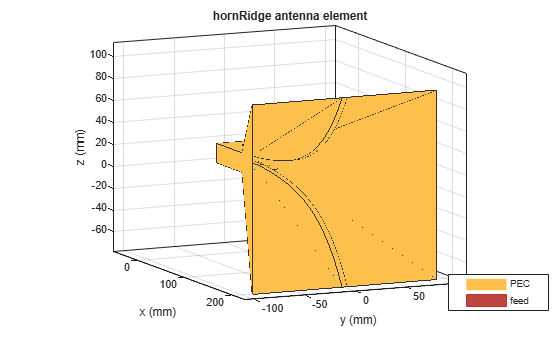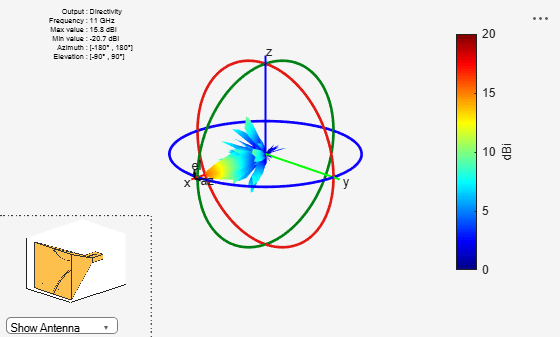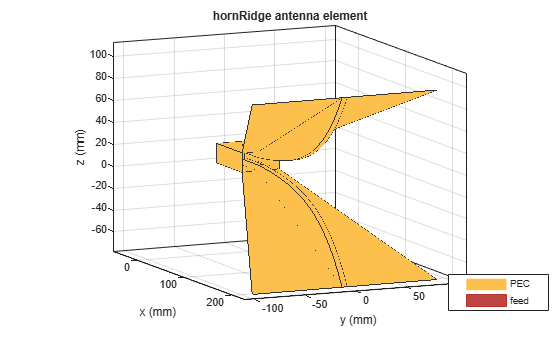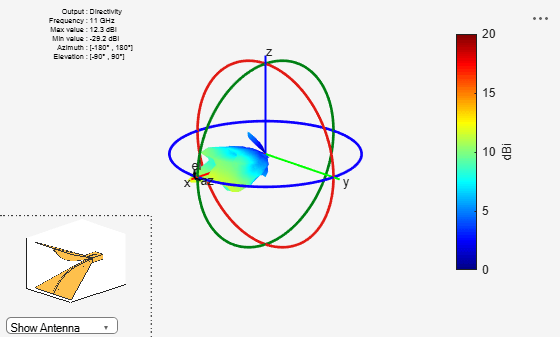Main Content

# hornRidge

Create double-ridged rectangular horn

## Description

The `hornRidge` object creates a double-ridged horn antenna with the default dimensions chosen for an operating frequency range of 10 GHz-12 GHz.Ridged horn antennas are commonly used in electromagnetic interference and compatibility applications for generating electromagnetic fields. These antennas are also used in radio astronomy or radar cross-section (RCS) measurements.

## Creation

### Syntax

``ant = hornRidge``
``ant = hornRidge(Name,Value)``

### Description

example

````ant = hornRidge` creates a double-ridged horn antenna with the default dimensions chosen for an operating frequency range of 10 GHz-12 GHz.```

example

````ant = hornRidge(Name,Value)` sets properties using one or more name-value pairs. For example, ```ant = hornRidge('FlareLength',178.38e-3)``` creates a ridged horn antenna object with a flare length of 178.39 millimeters.```

## Properties

expand all

Number of flares, specified as `0`, `2`, or `4`. Specify zero if you do not want any flares.

Example: `'NumFlares',2`

Example: `ant.NumFlares = 2`

Data Types: `double`

Length of the flare, specified as a nonnegative scalar in meters.

Example: `'FlareLength',0.2760`

Example: `ant.FlareLength = 0.2760`

Data Types: `double`

Width of the flare, specified as a nonnegative scalar in meters.

Example: `'FlareWidth',0.3760`

Example: `ant.FlareWidth = 0.3760`

Data Types: `double`

Height of the flare, specified as a nonnegative scalar in meters.

Example: `'FlareHeight',0.2560`

Example: `ant.FlareHeight = 0.2560`

Data Types: `double`

Length of the waveguide, specified as a nonnegative scalar in meters.

Example: `'Length',0.0676`

Example: `ant.Length = 0.0676`

Data Types: `double`

Width of the waveguide, specified as a nonnegative scalar in meters.

Example: `'Width',0.0476`

Example: `ant.Width = 0.0476`

Data Types: `double`

Height of the waveguide, specified as a nonnegative scalar in meters.

Example: `'Height',0.0340`

Example: `ant.Height = 0.0340`

Data Types: `double`

Length of the waveguide ridge, specified as a nonnegative scalar in meters.

Example: `'RidgeLength',0.0276`

Example: `ant.RidgeLength = 0.0276`

Data Types: `double`

Width of the waveguide ridge, specified as a nonnegative scalar in meters.

Example: `'RidgeWidth',0.0040`

Example: `ant.RidgeWidth = 0.0040`

Gap between the two ridges of the waveguide, specified as a nonnegative scalar in meters.

Example: `'RidgeGap',0.0060`

Example: `ant.RidgeGap = 0.0060`

Data Types: `double`

Radius of the feeding hole, specified as a nonnegative scalar in meters.

Example: `'FeedHoleRadius',0.0008`

Example: `ant.FeedHoleRadius = 0.0008`

Data Types: `double`

Width of the feed, specified as a nonnegative scalar in meters.

Example: `'FeedWidth',0.0002`

Example: `ant.FeedWidth = 0.0002`

Data Types: `double`

Signed distance from the closed end of the waveguide, specified as a two-element vector with each element unit in meters.

Example: `'FeedOffset',[-0.00626 0]`

Example: `ant.FeedOffset = [-0.00626,0]`

Data Types: `double`

Type of the metal used as a conductor, specified as a metal material object. You can choose any metal from the `MetalCatalog` or specify a metal of your choice. For more information, see `metal`. For more information on metal conductor meshing, see Meshing.

Example: ```m = metal('Copper'); 'Conductor',m```

Example: ```m = metal('Copper'); ant.Conductor = m```

Lumped elements added to the antenna feed, specified as a `lumpedElement` object. You can add a load anywhere on the surface of the antenna. By default, the load is at the feed. For more information, see `lumpedElement`.

Example: `'Load',lumpedElement`, where `lumpedElement` is load added to the antenna feed.

Example: `ant.Load = lumpedElement('Impedance',75)`

Tilt angle of the antenna, specified as a scalar or vector with each element unit in degrees. For more information, see Rotate Antennas and Arrays.

Example: `'Tilt',90`

Example: `ant.Tilt = 90`

Example: `'Tilt',[90 90]`,`'TiltAxis',[0 1 0;0 1 1]` tilts the antenna at 90 degrees about the two axes defined by the vectors.

Note

The `wireStack` antenna object only accepts the dot method to change its properties.

Data Types: `double`

Tilt axis of the antenna, specified as:

• Three-element vector of Cartesian coordinates in meters. In this case, each coordinate in the vector starts at the origin and lies along the specified points on the X-, Y-, and Z-axes.

• Two points in space, each specified as three-element vectors of Cartesian coordinates. In this case, the antenna rotates around the line joining the two points in space.

• A string input describing simple rotations around one of the principal axes, 'X', 'Y', or 'Z'.

For more information, see Rotate Antennas and Arrays.

Example: `'TiltAxis',[0 1 0]`

Example: `'TiltAxis',[0 0 0;0 1 0]`

Example: `ant.TiltAxis = 'Z'`

Note

The `wireStack` antenna object only accepts the dot method to change its properties.

Data Types: `double`

## Object Functions

 `show` Display antenna or array structure; display shape as filled patch `axialRatio` Axial ratio of antenna `beamwidth` Beamwidth of antenna `charge` Charge distribution on metal or dielectric antenna or array surface `current` Current distribution on metal or dielectric antenna or array surface `design` Design prototype antenna or arrays for resonance at specified frequency `efficiency` Radiation efficiency of antenna `EHfields` Electric and magnetic fields of antennas; Embedded electric and magnetic fields of antenna element in arrays `impedance` Input impedance of antenna; scan impedance of array `mesh` Mesh properties of metal or dielectric antenna or array structure `meshconfig` Change mesh mode of antenna structure `optimize` Optimize antenna or array using SADEA optimizer `pattern` Radiation pattern and phase of antenna or array; Embedded pattern of antenna element in array `patternAzimuth` Azimuth pattern of antenna or array `patternElevation` Elevation pattern of antenna or array `returnLoss` Return loss of antenna; scan return loss of array `sparameters` S-parameter object `vswr` Voltage standing wave ratio of antenna

## Examples

collapse all

Create and view a default double ridged horn antenna.

`ant = hornRidge`
```ant = hornRidge with properties: NumFlares: 4 FlareLength: 0.1784 FlareWidth: 0.1834 FlareHeight: 0.1732 Length: 0.0538 Width: 0.0370 Height: 0.0177 RidgeLength: 0.0370 RidgeWidth: 0.0050 RidgeGap: 0.0070 FeedHoleRadius: 5.0000e-04 FeedWidth: 1.0000e-04 FeedOffset: [-0.0076 0] Conductor: [1x1 metal] Tilt: 0 TiltAxis: [1 0 0] Load: [1x1 lumpedElement] ```
`show(ant)`Plot the radiation pattern of the antenna at 11 GHz.

```p = PatternPlotOptions('MagnitudeScale',[0 20]); figure; pattern(ant,11e9,'patternOptions',p);```Create and view a ridged horn antenna with 2 flares.

`ant = hornRidge('NumFlares',2)`
```ant = hornRidge with properties: NumFlares: 2 FlareLength: 0.1784 FlareWidth: 0.1834 FlareHeight: 0.1732 Length: 0.0538 Width: 0.0370 Height: 0.0177 RidgeLength: 0.0370 RidgeWidth: 0.0050 RidgeGap: 0.0070 FeedHoleRadius: 5.0000e-04 FeedWidth: 1.0000e-04 FeedOffset: [-0.0076 0] Conductor: [1x1 metal] Tilt: 0 TiltAxis: [1 0 0] Load: [1x1 lumpedElement] ```
`show(ant)`Plot the radiation pattern of the antenna at 11 GHz.

`p = PatternPlotOptions('MagnitudeScale',[0 20])`
```p = PatternPlotOptions with properties: Transparency: 1 SizeRatio: 0.9000 MagnitudeScale: [0 20] AntennaOffset: [0 0 0] ```
```figure; pattern(ant,11e9,'patternOptions',p)```## See Also

### Topics

Introduced in R2020b

## Support

#### Hybrid Beamforming for Massive MIMO Phased Array Systems

Download the white paper